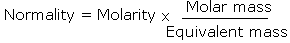Question & Answer
QUESTION

# What is the relation between normality and molarity of a solution ?

ANSWER

Normality and Molarity are related as:"Have a doubt going on your head? Download Vedantu's Instant Doubt Solving App now and get all your doubts cleared by our experts."

For acids

Normality = Molarity x Basicity

where basicity is the number of H+ ions a molecule of an acid can give

For bases

Normality = Molarity x Acidity

where acidity is the number of OH- ions a molecule of a base can give.